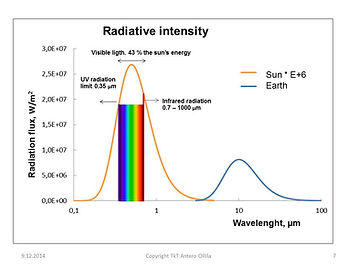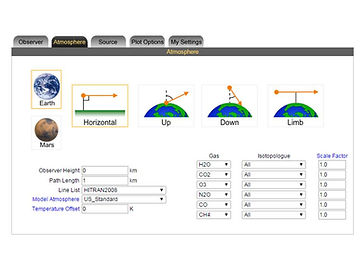## The scientific methodsSpectral calculation analysis

The author has used spectral calculation method in analysing the warming effects of greenhouse (GH) gases. This method is the only available method for this kind of analyses. It is impossible to carry out any trials with the atmosphere. On the other hand, the concentration changes of the GH gases are so small that the warming effects of the concentration changes are impossible to measure through temperature changes or through radiation changes.

That is why the only available method is the spectral analysis. Also, IPCC has utilized this method in calculating the warming effects of GH gases. When a researcher applies spectral analysis, it can be done only if a climate model - a simple or a more complicated one - is available. The differences between the different results are based on the applied climate models - especially water content and humidity conditions - and not the spectral analysis itself.

Spectral calculation tool

The author has utilized a spectral calculation tool prepared and maintained by GATS Inc., which is available on the Internet. This tool is quite flexible and the most accurate absorption line lists for the common GH gas molecules are available.The official name of this spectral calculation tool is the Spectral Calculator.Molecular spectral analysis

Molecules emit and absorb radiation only at certain frequencies or wavenumbers, thereby creating changes in their quantum energy levels. This produces a unique spectrum for each gas molecule species. According to Kirchhoff’s law, the absorptivity is the compliment of transmittance, which eq. 1 below shows:

Absorptivity = 1- transmittance     (1)

The Spectral Calculator tool produces the transmittance values only; the absorptivity values can easily be calculated using eq.1. The absorption lines appear as dips in the transmittance spectrum lines. Each line has a width and a depth, which are determined by the quantum mechanical properties of the molecule in question.

The spectrum of a gas molecule can be calculated using the basic physical laws, but it is not accurate enough for practical calculations. The collection of line parameters for a group of absorption lines is called a line list; the list has been derived by fitting laboratory spectra measured in various conditions. In this way, each absorption line is specified by a set of parameters. Using this data, an absorption line can be calculated for a given concentration, temperature and pressure. HITRAN is probably the most comprehensive and commonly used line list for atmospheric applications and it is available when using the SpectralCalc tool.

In order to simulate the transmittance spectrum of a gas mixture over a given spectral range, the absorption lines of each gas must be calculated. The complete spectrum is the product of the individual, absorption-line spectra. The individual line spectra cannot be directly summarized in the case of overlapping frequencies.

The algorithms that calculate molecular spectra in this way are known as line-by-line models, and they produce the most accurate values for molecular absorption. The Spectral Calculator tool uses line-by-line modelling for the molecular transmission and emission calculations. In actual calculations SpectralCalc uses LinepakTM algorithms, which Gordley et al. have described in detail. Many international research institutes, including NASA, use LinepakTM software for spectral calculations, which proves the correctness and validity of Spectral Calculator.Atmospheric spectral calculations

Calculating the spectrum in a complicated path, like through the planetary atmosphere, requires special arrangements. Spectral Calculator divides the path into segments, which are approximated by constant temperature, pressure and gas concentrations. The transmittance spectrum of the whole path is the product of individual spectra of the segments. These calculations may become computationally very intensive and, therefore, SpectralCalc has been designed to maximize efficiency without losing accuracy.

The Spectral Calculator tool offers an opportunity for individual researchers to calculate and analyze the GH phenomenon. Atmospheric path calculations are possible from a minimum height of 1 km to a maximum height of 600 km. Six different atmospheric models are readily available: standard, tropical, mid-latitude summer, mid-latitude winter, polar summer and polar winter. Users can modify the GH concentration profiles by scale factors and the temperature by offset changes. Both absorption and transmittance can be calculated.

The author has used the Spectral Calculator tool to verify some basic features of the GH phenomenon and especially the effects of GH gas concentration changes. Even though Spectral Calculator is a very powerful calculation tool, a user cannot calculate the transmission or absorption spectrum, for example in the range of 4-100 micrometres (µm), with one calculation operation only. The author calculated the absorption values in the ranges of 4-5, 5-6, 6-7, 7-10, 10-15, 15-20, 20-30 and 30-100 µm in an air column of 11 km. Each calculation produced a list of transmission and wavelength values varying from 403 to 564 points. The absorption of radiation energy for each wavelength was calculated by multiplying the absorption values by the calculated radiance values emitted by the Earth’s surface at a given temperature.

Two test runs were carried out for checking the correctness of radiation absorption procedures of integration. By selecting the transmission value as zero and the temperature as 255.2 K, the total absorption in an air column of 11 km was 238.61 Wm-2. Spectral Calculator’s black body calculator for the same temperature gave a reading of 240.52 Wm-2, which indicates 0.79% accuracy. The Spectral Calculator tool does not recalculate the temperature profile if there is radiation absorption in the atmosphere that causes a temperature change. This phenomenon causes a small error in calculations. It should be noticed that the temperature increase is very small having also a very small effect on the absorption values.

Accuracy of the spectral analysis method

The accuracy of spectral calculations can be checked using the real atmosphere conditions. The two studies show that the CO2 absorption in dry and clear atmosphere conditions that generally the calculation accuracy is below 1 %.

However the question of water vapor continuum is still unclear. The continuum means that the strong absorption bands of water have effects also on the neighboring bands. The continuum effects have been estimated by semi-empirical formulas in the spectral calculation tools. Spectral Calculator does not use water continuum effects in its atmospheric path calculations.

There is a simple way to check the accuracy of the atmospheric spectral calculations carried out by Spectral Calculator. In the clear sky conditions according to the Kirchhoff’s rule, the LW downward flux Ed emitted by the atmosphere should be the same as the total absorption Ag due to the absorption caused by the GH gases. According to the synthesis analysis of Stephens et al. , the measured value of downward fluxes vary between 309.2 - 326 Wm-2 in 13 independent studies the average value being 314.2 Wm-2. The author has calculated the flux value of Ag to be 310.9 Wm-2 using the Spectral Calculator’s formula for water vapor. The conclusion is that Spectral Calculator gives results, which are near to the measured values of the real atmosphere and the difference is in average about 1.0 %. This difference is well inside the error margin of ±10 Wm.2 estimated to be the accuracy of measured LW fluxes .

Some researchers apply water continuum calculation formula in their analyses. It means that the absorption by water is stronger and the absorption by CO2 is weaker. In this respect the results of my papers are "conservative" in respect to CO2, which means that the effects of CO2 are not certainly too weak.

References:

   G. L. Stephens, M. Wild, P. W. Stackhouse Jr., T. L’Ecuyer, S. Kato and D. S. Henderson, “The global character of the flux of downward longwave radiation, ” Journal of Climate, vol. 25, 2012,  pp. 2329-2340.

   D. D. Turner, D.C. Tobin, S. A. Clough, P. D. Brown, R. G. Ellingson, E. J. Mlawer, R. O. Knuteson , H. E. Revercomb, T. R. Shippert, W. L. Smith and M. W. Shephard, “The QME AERI LBLRTM: A closure experiment for downwelling high spectral resolution infrared radiance,” Journal of Atmospheric Sciences, vol. 61, 2004, pp. 2657-2675.